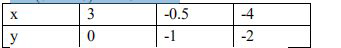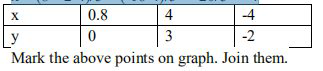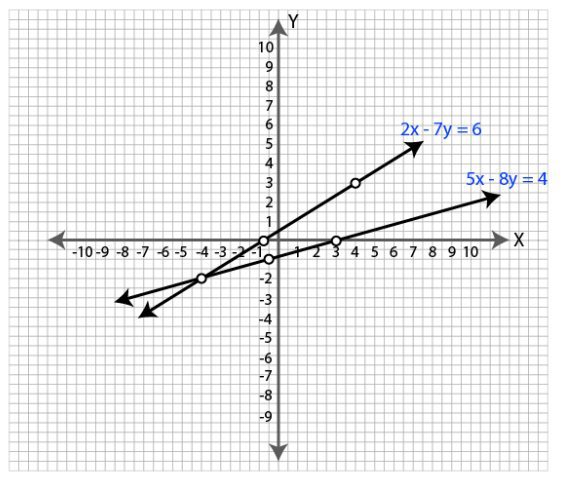Ml aggarwal solutionsOur top 5% students will be awarded a special scholarship to Lido.
Set your child up for success with Lido, book a class today!

. Solve the following pair of equations graphically. Plot at least 3 points for each straight line 2x -7y = 6, 5x

-8y = -4.Looking to do well in your science exam ? Learn from an expert tutor. Book a free class!

2x-7y = 6 ….(i)

2x = 7y+6

x = (7y+6)/2

when y = 0

x = (7×0+6)/2 = 6/2 = 3

when y = -1

x = (7×-1+6)/2 = -1/2 = -0.5

when y = -2

x = (7×-2+6)/2 = -8/2 = -4Mark the above points on the graph. Join them.

5x = 8y-4

x = (8y-4)/5

when y = 0

x = (8×0-4)/5 = -4/5 = 0.8

when y = 3

x = (8×3-4)/5 = (24-4)/5 = 20/5 = 4

when y = -2

x = (8×-2-4)/5 = (-16-4)/5 = -20/5 = -4

5x-8y = -4 …(ii)It is clear from the graph that the two lines intersect at (-4,-2).

So the solution of the given equations are x = -4 and y = -2.

Our top 5% students will be awarded a special scholarship to Lido.Lido

Courses

Teachers

Book a Demo with us

Syllabus

Science
English
Coding

Terms & Policies

NCERT Question Bank

Maths
Science

Selina Question Bank

Maths
Physics
Biology

Allied Question Bank

Chemistry